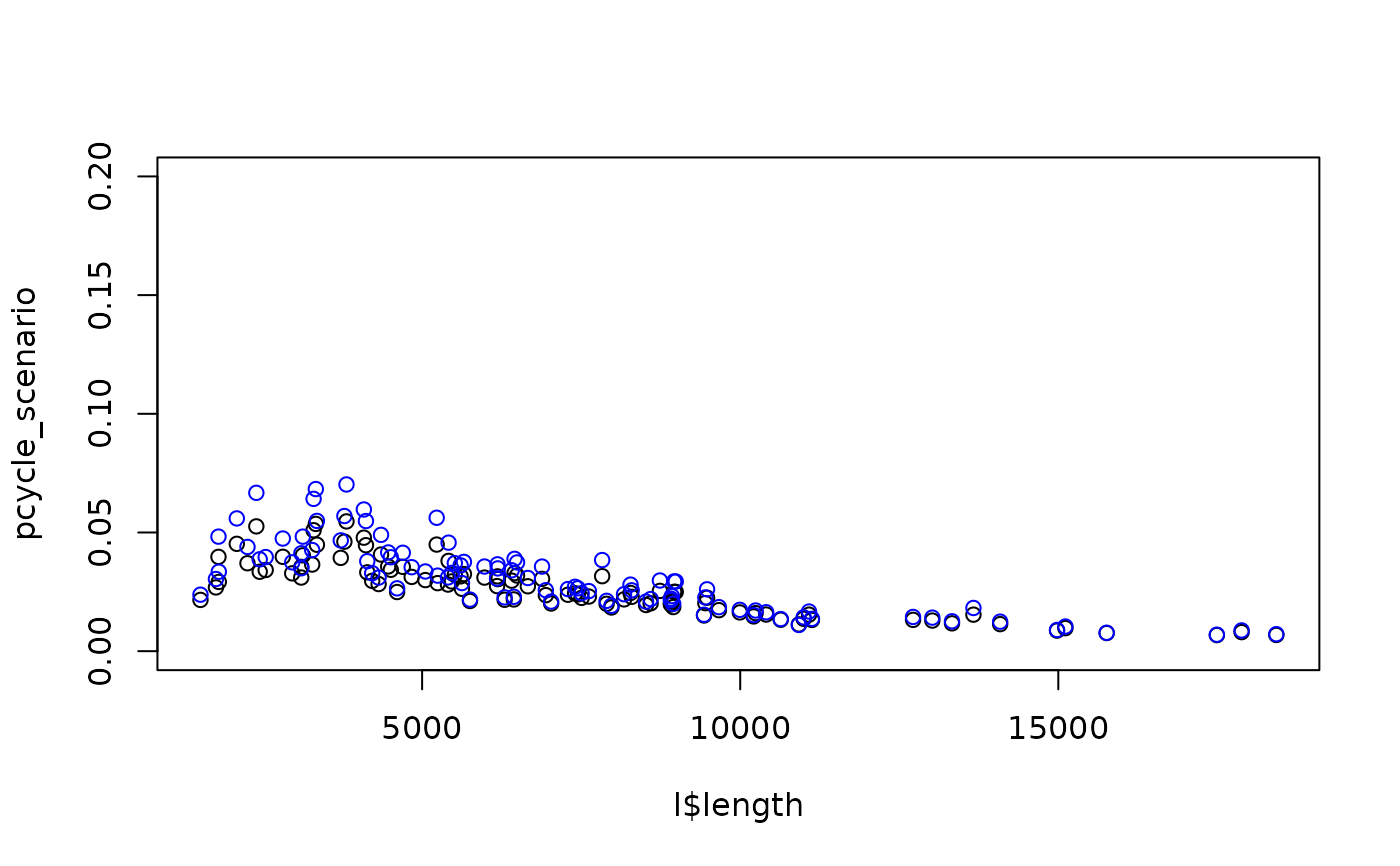Uptake model that takes distance and hilliness and returns a percentage of trips that could be made by cycling along a desire line under scenarios of change. Source: appendix of pct paper, hosted at: www.jtlu.org which states that: "To estimate cycling potential,the Propensity to Cycle Tool (PCT) was designed to use the best available geographically disaggregated data sources on travel patterns."

uptake_pct_govtarget(
distance,
alpha = -3.959,
d1 = -0.5963,
d2 = 1.866,
d3 = 0.00805,
h1 = -0.271,
i1 = 0.009394,
i2 = -0.05135,
verbose = FALSE
)

uptake_pct_govtarget_2020(
distance,
alpha = -4.018,
d1 = -0.6369,
d2 = 1.988,
d3 = 0.008775,
h1 = -0.2555,
h2 = -0.78,
i1 = 0.02006,
i2 = -0.1234,
verbose = FALSE
)

uptake_pct_godutch_2020(
distance,
alpha = -4.018 + 2.55,
d1 = -0.6369 - 0.08036,
d2 = 1.988,
d3 = 0.008775,
h1 = -0.2555,
h2 = -0.78,
i1 = 0.02006,
i2 = -0.1234,
verbose = FALSE
)

uptake_pct_ebike_2020(
distance,
alpha = -4.018 + 2.55,
d1 = -0.6369 - 0.08036 + 0.05509,
d2 = 1.988,
d3 = 0.008775 - 0.000295,
h1 = -0.2555 + 0.1812,
h2 = -0.78,
i1 = 0.02006,
i2 = -0.1234,
verbose = FALSE
)

uptake_pct_govtarget_school2(
distance,
alpha = -7.178,
d1 = -1.87,
d2 = 5.961,
h1 = -0.529,
h2 = -0.63,
verbose = FALSE
)

uptake_pct_godutch_school2(
distance,
alpha = -7.178 + 3.574,
d1 = -1.87 + 0.3438,
d2 = 5.961,
h1 = -0.529,
h2 = -0.63,
verbose = FALSE
)

## Arguments

distance

Vector distance numeric values of routes in km (switches to km if more than 100).

Vector gradient numeric values of routes.

alpha

The intercept

d1

Distance term 1

d2

Distance term 2

d3

Distance term 3

h1

Hilliness term 1

i1

Distance-hilliness interaction term 1

i2

Distance-hilliness interaction term 2

verbose

Print messages? FALSE by default.

h2

Hilliness term 2

## Details

The functional form of the cycling uptake model used in the PCT is as follows: (Source: npct.github.io)

logit (pcycle) = -3.959 +   # alpha
(-0.5963 * distance) +    # d1
(1.866 * distancesqrt) +  # d2
(0.008050 * distancesq) + # d3
(-0.2710 * gradient) +    # h1
(0.009394 * distance * gradient) +  # i1
(-0.05135 * distancesqrt *gradient) # i2

pcycle = exp ([logit (pcycle)]) / (1 + (exp([logit(pcycle)])


uptake_pct_govtarget_2020() and uptake_pct_godutch_2020() approximate the uptake models used in the updated 2020 release of the PCT results.

If the distance parameter is greater than 100, it is assumed that it is in m. If for some reason you want to model cycling uptake associated with trips with distances of less than 100 m, convert the distances to km first.

## Examples

distance = 15
logit_pcycle = -3.959 + # alpha
(-0.5963 * distance) + # d1
(1.866 * sqrt(distance)) + # d2
(0.008050 * distance^2) + # d3
(-0.2710 * gradient) + # h1
(0.009394 * distance * gradient) + # i1
(-0.05135 * sqrt(distance) * gradient) # i2
boot::inv.logit(logit_pcycle)
#>  0.0107377
uptake_pct_govtarget(15, 2)
#>  0.0107377
l = routes_fast_leeds
pcycle_scenario = uptake_pct_govtarget(l$length, l$av_incline)
pcycle_scenario_2020 = uptake_pct_govtarget_2020(l$length, l$av_incline)
plot(l$length, pcycle_scenario, ylim = c(0, 0.2)) points(l$length, pcycle_scenario_2020, col = "blue")# compare with published PCT data:
l_pct_2020 = get_pct_lines(region = "isle-of-wight")
# test for another region:
# l_pct_2020 = get_pct_lines(region = "west-yorkshire")
l_pct_2020$rf_avslope_perc[1:5] #>  0.52 0.30 0.32 0.46 0.49 l_pct_2020$rf_dist_km[1:5]
#>  14.6 11.7 11.3 11.7 12.1
govtarget_slc = uptake_pct_govtarget(
distance = l_pct_2020$rf_dist_km, gradient = l_pct_2020$rf_avslope_perc
) * l_pct_2020$all + l_pct_2020$bicycle
govtarget_slc_2020 = uptake_pct_govtarget_2020(
distance = l_pct_2020$rf_dist_km, gradient = l_pct_2020$rf_avslope_perc
) * l_pct_2020$all + l_pct_2020$bicycle
mean(l_pct_2020$govtarget_slc) #>  1.233474 mean(govtarget_slc) #>  1.171526 mean(govtarget_slc_2020) #>  1.233669 godutch_slc = uptake_pct_godutch( distance = l_pct_2020$rf_dist_km,
gradient = l_pct_2020$rf_avslope_perc ) * l_pct_2020$all + l_pct_2020$bicycle godutch_slc_2020 = uptake_pct_godutch_2020( distance = l_pct_2020$rf_dist_km,
gradient = l_pct_2020$rf_avslope_perc ) * l_pct_2020$all + l_pct_2020$bicycle mean(l_pct_2020$dutch_slc)
#>  3.574052
mean(godutch_slc)
#>  3.828446
mean(godutch_slc_2020)
#>  4.191658
# Take an origin destination (OD) pair between an LSOA centroid and a
# secondary school. In this OD pair, 30 secondary school children travel, of
# whom 3 currently cycle. The fastest route distance is 3.51 km and the